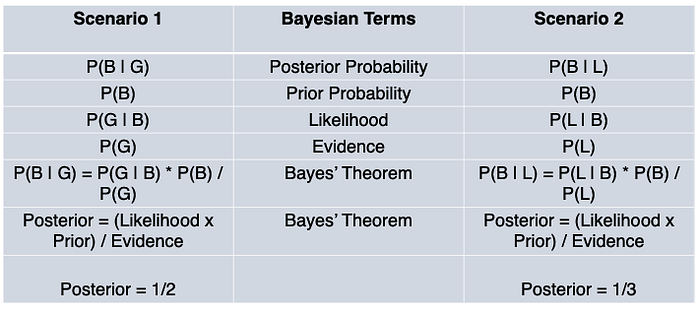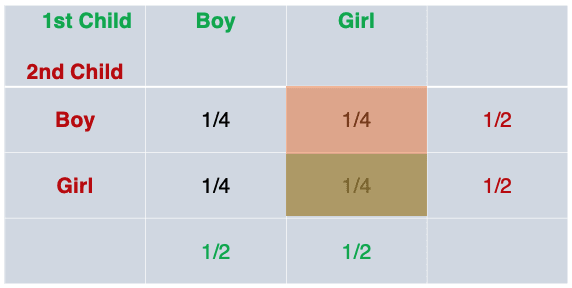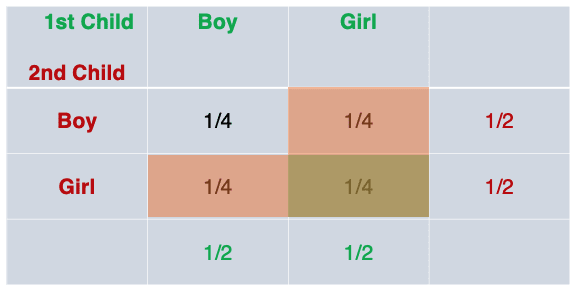# Bayes' Theorem: Concepts and Code

## Building on our understanding of conditional probability we'll get into Bayes' Theorem

### Overview

This post is a in continuation of my coverage of Data Science from Scratch by Joel Grus.

It picks up from the previous post, so be sure to check that out for proper context.

Building on our understanding of conditional probability we'll get into Bayes' Theorem. We'll spend some time understanding the concept before we implement an example in code.

### Bayes Theorem

Previously, we established an understanding of conditional probability, but building up with marginal and joint probabilities. We explored the conditional probabilities of two outcomes:

#### Outcome 1: What is the probability of the event “both children are girls” (B) conditional on the event “the older child is a girl” (G)?

The probability for outcome one is roughly 50% or (1/2).

#### Outcome 2: What is the probability of the event “both children are girls” (B) conditional on the event “at least one of the children is a girl” (L)?

The probability for outcome two is roughly 33% or (1/3).

Bayes' Theorem is simply an alternate way of calculating conditional probability.

Previously, we used the joint probability to calculate the conditional probability.

### Outcome 1

Here's the conditional probability for outcome 1, using a joint probability:

• P(G) = 'Probability that first child is a girl' (1/2)
• P(B) = 'Probability that both children are girls' (1/4)
• P(B|G) = P(B,G) / P(G)
• P(B|G) = (1/4) / (1/2) = 1/2 or roughly 50%

Technically, we can't use joint probability because the two events are not independent.

To clarify, the probability of the older child being a certain gender and the probability of the younger child being a certain gender is independent, but `P(B|G)` the 'probability of both child being a girl' and 'the probability of the older child being a girl' are not independent; and hence we express it as a conditional probability.

So, the joint probability of `P(B,G)` is just event B,`P(B)`.

Here's an alternate way to calculate the conditional probability (without joint probability):

• `P(B|G) = P(G|B) * P(B) / P(G)` This is Bayes Theorem
• P(B|G) = 1 * (1/4) / (1/2)
• P(B|G) = (1/4) * (2/1)
• P(B|G) = 1/2 = 50%

note: P(G|B) is 'the probability that the first child is a girl, given that both children are girls is a certainty (1.0)'

The reverse conditional probability, can also be calculated, without joint probability:

#### What is the probability of the older child being a girl, given that both children are girls?

• `P(G|B) = P(B|G) * P(G) / P(B)` This is Bayes Theorem (reverse case)
• P(G|B) = (1/2) * (1/2) / (1/4)
• P(G|B) = (1/4) / (1/4)
• P(G|B) = 1 = 100%

This is consistent with what we already derived above, namely that P(G|B) is a certainty (probability = 1.0), that the older child is a girl, given that both children are girls.

We can point out two additional observations / rules:

1. While, joint probabilities are symmetrical: P(B,G) == P(G,B),
2. Conditional probabilities are not symmetrical: P(B|G) != P(G|B)

### Bayes' Theorem: Alternative Expression

Bayes Theorem is a way of calculating conditional probability without the joint probability, summarized here:

• `P(B|G) = P(G|B) * P(B) / P(G)` This is Bayes Theorem
• `P(G|B) = P(B|G) * P(G) / P(B)` This is Bayes Theorem (reverse case)

You'll note that `P(G)` is the denominator in the former, and `P(B)` is the denominator in the latter.

#### What if, for some reasons, we don't have access to the denominator?

We could derive both `P(G)` and `P(B)` in another way using the `NOT` operator:

• P(G) = P(G,B) + P(G,not B) = P(G|B) _ P(B) + P(G|not B) _ P(not B)
• P(B) = P(B,G) + P(B,not G) = P(B|G) _ P(G) + P(B|not G) _ P(not G)

Therefore, the alternative expression of Bayes Theorem for the probability of both children being girls, given that the first child is a girl ( P(B|G) ) is:

• P(B|G) = P(G|B) _ P(B) / ( P(G|B) _ P(B) + P(G|not B) * P(not B) )
• P(B|G) = 1 _ 1/4 / (1 _ 1/4 + 1/3 * 3/4)
• P(B|G) = 1/4 / (1/4 + 3/12)
• P(B|G) = 1/4 / 2/4 = 1/4 * 4/2
• P(B|G) = 1/2 or roughly 50%

We can check the result in code:

``````def bayes_theorem(p_b, p_g_given_b, p_g_given_not_b):
# calculate P(not B)
not_b = 1 - p_b
# calculate P(G)
p_g = p_g_given_b * p_b + p_g_given_not_b * not_b
# calculate P(B|G)
p_b_given_g = (p_g_given_b * p_b) / p_g
return p_b_given_g

#P(B)
p_b = 1/4
# P(G|B)
p_g_given_b = 1
# P(G|notB)
p_g_given_not_b = 1/3
# calculate P(B|G)
result = bayes_theorem(p_b, p_g_given_b, p_g_given_not_b)
# print result
print('P(B|G) = %.2f%%' % (result * 100))
``````

For the probability that the first child is a girl, given that both children are girls ( P(G|B) ) is:

• P(G|B) = P(B|G) _ P(G) / ( P(G|B) _ P(G) + P(B|not G) * P(not G) )
• P(G|B) = 1/2 _ 1/2 / ((1/2 _ 1/2) + (0 * 1/2))
• P(G|B) = 1/4 / 1/4
• P(G|B) = 1

Let's unpack Outcome 2.

### Outcome 2

Outcome 2: What is the probability of the event “both children are girls” (B) conditional on the event “at least one of the children is a girl” (L)?

The probability for outcome two is roughly 33% or (1/3).

We'll go through the same process as above.

We could use joint probability to calculate the conditional probability. As with the previous outcome, the joint probability of `P(B,G)` is just event B,`P(B)`.

• P(B|L) = P(B,L) / P(L) = 1/3

Or, we could use Bayes' Theorem to figure out the conditional probability without joint probability:

• P(B|L) = P(L|B) * P(B) / P(L)
• P(B|L) = (1 * 1/4) / (3/4)
• P(B|L) = 1/3

And, if there's no `P(L)`, we can calculate that indirectly, also using Bayes' Theorem:

• P(L) = P(L|B) _ P(B) + P(L|not B) _ P(not B)
• P(L) = 1 _ (1/4) + (2/3) _ (3/4)
• P(L) = (1/4) + (2/4)
• P(L) = 3/4

Then, we can use `P(L)` in the way Bayes' Theorem is commonly expressed, when we don't have the denominator:

• P(B|L) = P(L|B) _ P(B) / ( P(L|B) _ P(B) + P(L|not B) * P(not B) )
• P(B|L) = 1 * (1/4) / (3/4)
• P(B|L) = 1/3

Now that we've gone through the calculation for two conditional probabilities, `P(B|G)` and `P(B|L)`, using Bayes Theorem, and implemented code for one of the scenarios, let's take a step back and assess what this means.

### Bayesian Terminology

I think it's useful to understand that probability in general shines when we want to describe uncertainty and that Bayes' Theorem allows us to quantify how much the data we observe, should change our beliefs.We have two posteriors, `P(B|G)` and `P(B|L)`, both with equal priors and likelihood, but with different evidence.

Said differently, we want to know the 'probability that both children are girls`, given different conditions.

In the first case, our condition is 'the first child is a girl' and in the second case, our condition is 'at least one of the child is a girl'. The question is which condition will increase the probability that both children are girls?

Bayes' Theorem allows us to update our belief about the probability in these two cases, as we incorporate varied data into our framework.

What the calculations tell us is that the evidence that 'one child is a girl' increases the probability that both children are girls more than the other piece of evidence that 'at least one child is a girl' increases that probability.

And our beliefs should be updated accordingly.

At the end of the day, understanding conditional probability (and Bayes Theorem) comes down to counting. For our hypothetical scenarios, we only need one hand:

When we look at the probability table for outcome one, `P(B|G)`, we can see how the posterior probability comes out to 1/2:When we look at the probability table for outcome two, `P(B|L)`, we can see how the posterior probability comes out to 1/3:This is part of an ongoing series documenting my progress through Data Science from Scratch by Joel Grus:For more content on data science, machine learning, R, Python, SQL and more, find me on Twitter.